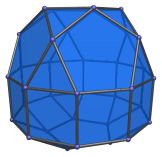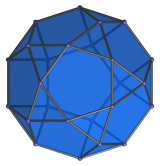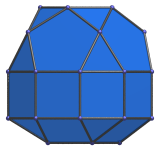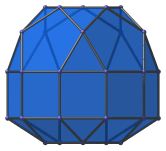# The Elongated Pentagonal Gyrocupolarotunda

The elongated pentagonal gyrocupolarotunda is the 41st Johnson solid (J41). It has 35 vertices, 70 edges, and 37 faces (15 equilateral triangles, 15 squares, 7 pentagons).The elongated pentagonal gyrocupolarotunda can be constructed by attaching a pentagonal rotunda and a pentagonal cupola to a decagonal prism. Or equivalently, inserting a decagonal prism between the rotunda and cupola parts of a pentagonal gyrocupolarotunda (J33). The gyro- in the name refers to how the top and bottom pentagons are rotated with respect to each other. If they are aligned with each other instead, the elongated pentagonal orthocupolarotunda (J40) is produced instead.

## Projections

Here are some views of the elongated pentagonal gyrocupolarotunda from various angles:

Projection DescriptionTop view.Front view.Side view.

## Coordinates

The Cartesian coordinates of the elongated pentagonal gyrocupolarotunda with edge length 2 are:

• (−√((10+2√5)/5), 0, 1+√((20+8√5)/5))
• (−√((5−√5)/10), ±φ, 1+√((20+8√5)/5))
• ( √((5+2√5)/5), ±1, 1+√((20+8√5)/5))
• ( √((20+8√5)/5), 0, 1+√((10+2√5)/5))
• (−√((25+11√5)/10), ±φ, 1+√((10+2√5)/5))
• ( √((5+√5)/10), ±φ2, 1+√((10+2√5)/5))
• (±√(3+4φ), ±1, ±1)
• (±√(2+φ), ±φ2, ±1)
• (0, ±2φ, ±1)
• (√((10+2√5)/5), 0, −(1+2√((3−φ)/5)))
• (√((5−√5)/10), ±φ, −(1+2√((3−φ)/5)))
• (−√((5+2√5)/5), ±1, −(1+2√((3−φ)/5)))

where φ=(1+√5)/2 is the Golden Ratio.

Last updated 03 Sep 2018.